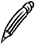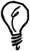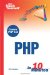# Numeric Functions

Let's take a look at some of the numeric functions available in PHP.

#### Rounding Numbers

There are three different PHP functions for rounding a decimal number to an integer.

You use ceil or floor to round a number up or down to the nearest integer, respectively. For example, ceil(1.3) returns 2, whereas floor(6.8) returns 6.Negative Rounding Note the way that negative numbers are rounded. The result of floor(-1.1) is-2the next lowest whole number numericallynot-1. Similarly, ceil(-2.5) returns -2.

To round a value to the nearest whole number, you use round. A fractional part under .5 will be rounded down, whereas .5 or higher will be rounded up. For example, round(1.3) returns 1, whereas round(1.5) returns 2.

The round function can also take an optional precision argument. The following example displays a value rounded to two decimal places:

` \$score = 0.535; echo round(\$score, 2); `

The value displayed is 0.54; the third decimal place being 5 causes the final digit to be rounded up.

You can also use round with a negative precision value to round an integer to a number of significant figures, as in the following example:

` \$distance = 2834; echo round(\$distance, -2); `

#### Comparisons

To find the smallest and largest of a group of numbers, you use min and max, respectively. These functions take two or more arguments and return the numerically lowest or highest element in the list, respectively.

This statement will display the larger of the two variables \$a and \$b:

` echo max(\$a, \$b); `

There is no limit to the number of arguments that can be compared. The following example finds the lowest value from a larger set of values:

` echo min(6, 10, 23, 3, 88, 102, 5, 44); `

Not surprisingly, the result displayed is 3.

#### Random Numbers

You use rand to generate a random integer, using your system's built-in random number generator. The rand function optionally takes two arguments that specify the range of numbers from which the random number will be picked.Random Limit The constant RAND_MAX contains the highest random number value that can be generated on your system. This value may vary between different platforms.

The following statement picks a random number between 1 and 10 and displays it:

` echo rand(1, 10); `

You can put this command in a script and run it a few times to see that the number changes each time it is run.

There is really no such thing as a computer-generated random number. In fact, numbers are actually picked from a very long sequence that has very similar properties to true random numbers. To make sure you always start from a different place in this sequence, you have to seed the random number generator by calling the srand function; no arguments are required.Random Algorithms PHP includes another random number generator, known as Mersenne Twister, that is considered to produce better random results than rand. To use this algorithm, you use the functions mt_rand and mt_srand.

#### Mathematical Functions

PHP includes many mathematical functions, including trigonometry, logarithms, and number base conversions. As you will rarely need to use these in a web environment, those functions are not covered in this book.

To find out about a function that performs a specific mathematical purpose, refer to the online manual at www.php.net/manual/en/ref.math.php.Sams Teach Yourself PHP in 10 Minutes
ISBN: 0672327627
EAN: 2147483647
Year: 2005
Pages: 151
Authors: Chris Newman

Similar book on Amazon

flylib.com © 2008-2017.
If you may any questions please contact us: flylib@qtcs.net